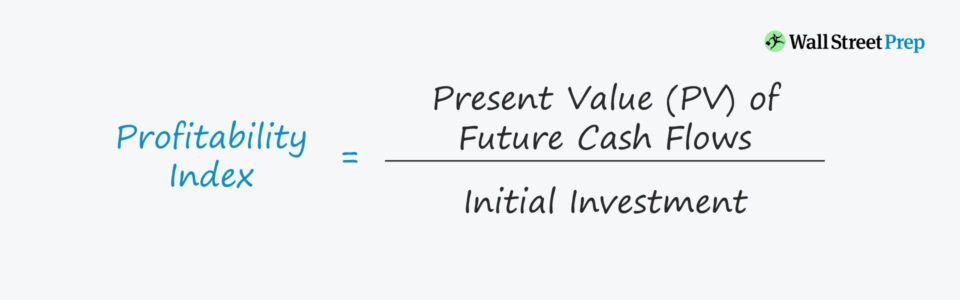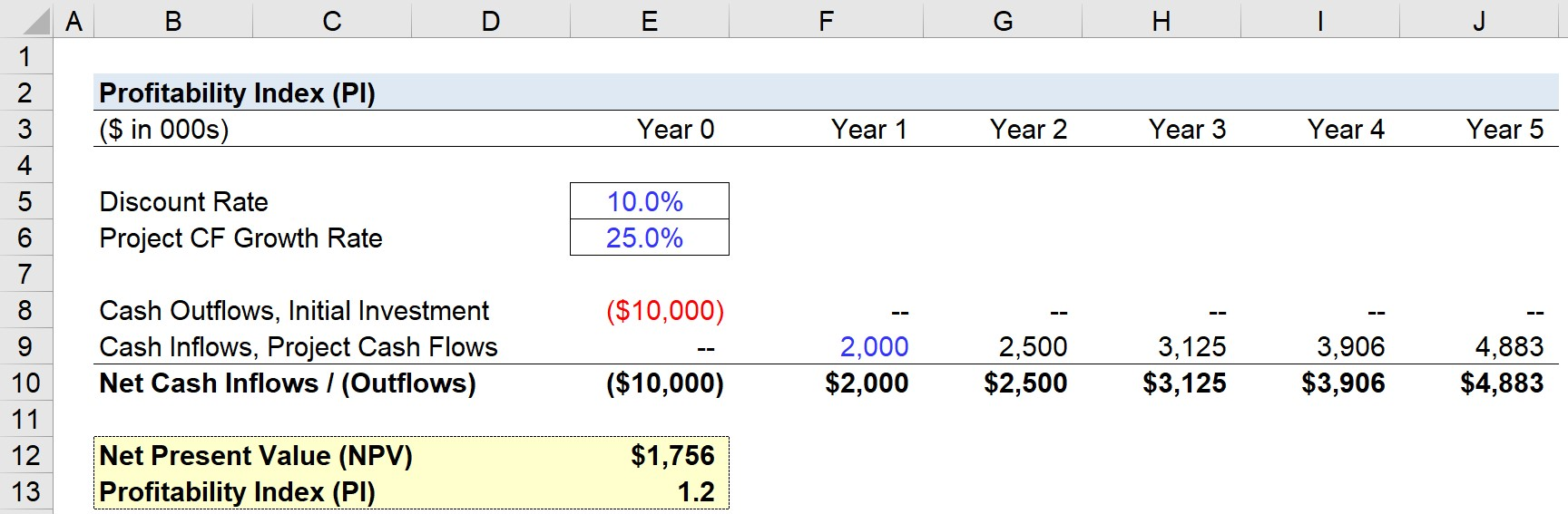Welcome to Wall Street Prep! Use code at checkout for 15% off.# Profitability Index (PI)

Guide to Understanding the Profitability Index## How to Calculate Profitability Index (Step-by-Step)

The profitability index ratio measures the monetary benefits (i.e. cash inflows) received for each dollar invested (i.e. cash outflow), with the cash flows discounted back to the present date.

More specifically, the PI ratio compares the present value (PV) of future cash flows received from a project to the initial cash outflow (investment) to fund the project.

## Profitability Index Formula

The formula for calculating the profitability index is as follows.

Profitability Index = Present Value of Future Cash Flows / Initial Investment

Another variation of the PI formula adds the initial investment to the net present value (NPV), which is then divided by the initial investment.

Profitability Index = (Net Present Value + Initial Investment) / Initial Investment

## How to Interpret Profitability Index (PI)

In corporate finance, the primary use case for the PI ratio is for ranking projects and capital investments.

The higher the PI ratio, the more attractive the proposed project is and the more likely it will be pursued.

For some general guidelines on interpreting the PI ratio:

• PI =1: Neutral/Acceptable
• PI >1: Approve Project
• PI <1: Reject Project

## Profitability Index vs. Net Present Value (NPV)

The profitability index (PI) and net present value (NPV) are two closely related metrics.

• If PI Ratio is >1, then NPV will be positive
• If PI Ratio is <1, then NPV will be negative

The major distinction between the two is that the profitability index depicts a “relative” measure of value whereas the net present value (NPV) represents an “absolute” measure of value.

With that said, for purposes of presenting a project or capital investment’s benefits on a per-dollar basis of the initial investment, the profitability index is more practical since it is standardized.

The PI metric can thereby be used for comparisons among different projects. By contrast, comparisons of NPV between projects are not always functional (i.e. non-standardized metric).

## Profitability Index Calculator – Excel Model Template

We’ll now move to a modeling exercise, which you can access by filling out the form below.Submitting...

## Step 1. Project Assumptions

Suppose we’re evaluating a proposed five-year project with the following assumptions.

• Discount Rate: 10%
• Project CF Growth Rate: 25%
• Initial Investment: –\$10,000,000
• Project Cash Flows (Year 1): \$2,000,000

The cost of funding the project is \$10 million, and the amount of cash flows generated in Year 1 is \$2 million, which will grow by a growth rate of 25% each year.

## Step 2. NPV Calculation

We can now calculate the net present value (NPV) of the project using the NPV function in Excel:

• NPV: “=NPV (10% Discount Rate, Range of Net Cash Inflows/Outflows)”
• NPV = \$1,756,382

In the subsequent step, we can now calculate the project’s PI given the NPV from the prior step.

## Step 3. Profitability Index Calculation Analysis

The PI formula will consist of two parts:

1. In the numerator, we’ll take the NPV and add back the initial investment.
2. In the denominator, we’ll link to the initial investment cell with a negative sign in front (so both the numerator and denominator are positive figures).

Therefore, the formula divides the present value (PV) of the project’s future cash flows by the initial investment.

• Profitability Index = (\$1,756,382 + \$10,000,000) / (\$10,000,000) = 1.2

In conclusion, the profitability index of our five-year project is 1.2, so the project seems likely to be accepted unless there are other projects with higher NPVs and profitability indices that are also under consideration.Step-by-Step Online Course

### Everything You Need To Master Financial Modeling

Enroll in The Premium Package: Learn Financial Statement Modeling, DCF, M&A, LBO and Comps. The same training program used at top investment banks.Inline FeedbacksLearn Financial Modeling Online

Everything you need to master financial and valuation modeling: 3-Statement Modeling, DCF, Comps, M&A and LBO.

X

The Wall Street Prep Quicklesson Series

7 Free Financial Modeling Lessons

Get instant access to video lessons taught by experienced investment bankers. Learn financial statement modeling, DCF, M&A, LBO, Comps and Excel shortcuts.